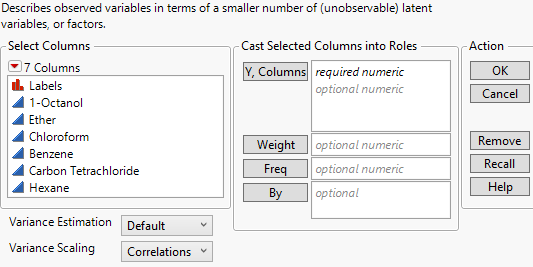Multivariate Methods > Factor Analysis > Launch the Factor Analysis Platform

Publication date: 06/16/2020

# Launch the Factor Analysis Platform

Launch the Factor Analysis platform by selecting Analyze > Multivariate Methods > Factor Analysis.

Figure 9.4 Factor Analysis Launch WindowY, Columns

The columns to be analyzed. These must have a Numeric data type.

Weight

A column containing a weight for each observation in the data table. A row is included in the analyses only when its value is greater than zero.

Freq

Assigns a frequency to each row in the analysis. This is useful when your data are summarized.

By

Produces a separate report for each level of the By variable. If more than one By variable is assigned, a separate report is produced for each possible combination of the levels of the By variables.

Variance Estimation

Lists the methods for estimating the variance-covariance matrix for the analysis. For more information about the methods, see The Multivariate Report.

Variance Scaling

Lists the scaling methods for performing the factor analysis.

Correlations

Default method that enables analysis on correlations.

Covariances

Enables the analysis on a weighted correlation matrix where the weights are the variables’ variances.

Unscaled

Enables the analysis of variables that are already centered or scaled.